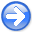Madagascar tutorialNext: Solution Up: Maurice: Tutorial Previous: Problem

1-D syntheticstep,smooth
Figure 8.
(a) 1-D synthetic to test edge-preserving smoothing. (b) Output of conventional triangle smoothing.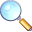To better understand the effect of smoothing, you decide to create a one-dimensional synthetic example shown in Figure 8a. The synthetic contains both sharp edges and random noise. The output of conventional triangle smoothing is shown in Figure 8b. We see an effect similar to the one in the real data example: random noise gets removed by smoothing at the expense of blurring the edges. Can you do better?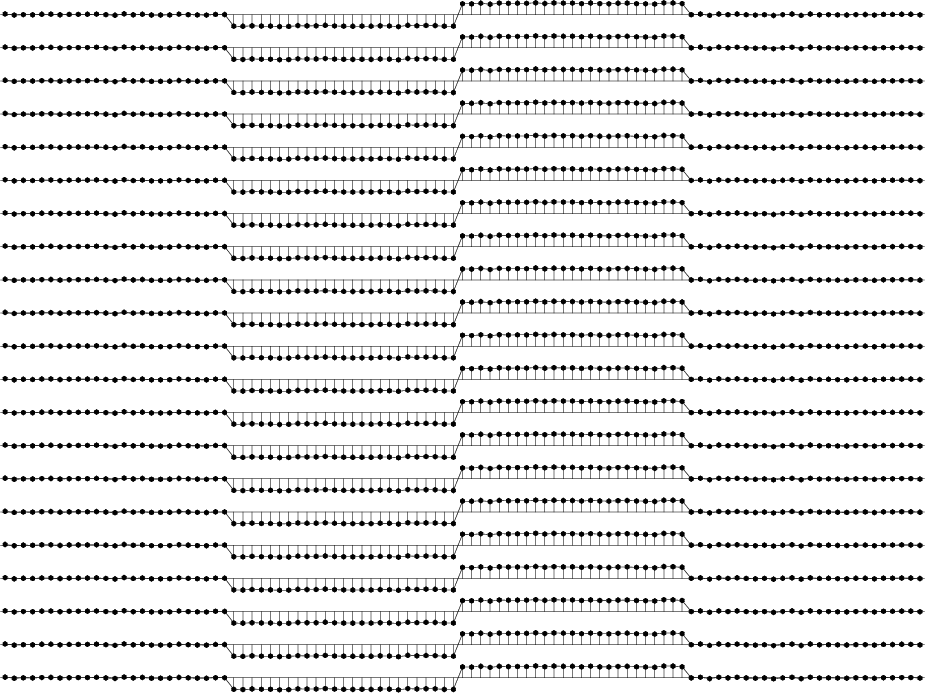spray,local
Figure 9.
(a) Input synthetic trace duplicated multiple times. (b) Duplicated traces shifted so that each data sample gets surrounded by its neighbors. The original trace is in the middle.To better understand what is happening in the process of smoothing, let us convert 1-D signal into a 2-D signal by first replicating the trace several times and then shifting the replicated traces with respect to the original trace (Figure 9). This creates a 2-D dataset, where each sample on the original trace is surrounded by samples from neighboring traces.

Every local filtering operation can be understood as stacking traces from Figure 9b multiplied by weights that correspond to the filter coefficients.

1. Change directory to the project directory
bash$cd ../local 2. Verify the claim above by running bash$ scons smooth.view smooth2.view

Open the SConstruct file in a text editor to verify that the first image is computed by sfsmooth and the second image is computed by applying triangle weights and stacking. To compare the two images by flipping between them, run
bash$sfpen Fig/smooth.vpl Fig/smooth2.vpl 3. Edit SConstruct to change the weight from triangle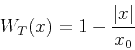(1) to Gaussian(2) Repeat the previous computation. Does the result change? What is a good value for? 4. Thinking about this problem, you invent an idea. Why not apply non-linear filter weights that would discriminate between points not only based on their distance from the center point but also on the difference in function values between the points. That is, instead of filtering by(3) whereis input,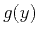is output, andis a linear weight, you decide to filter by(4) where andis a non-linear weight. Compare the two weights by running bash$ scons triangle.view similarity.view

The results should look similar to Figure 10.
5. The final output is Figure 11. By examining SConstruct, find how to reproduce this figure.
6. EXTRA CREDIT If you are familiar with programming in C, add 1-D non-local filtering as a new MADAGASCAR module sfnonloc. Ask the instructor for further instructions.triangle,similarity
Figure 10.
(a) Linear and stationary triangle weights. (b) Non-linear and non-stationary weights reflecting both distance between data points and similarity in data values.nlsmooth
Figure 11.
Output of non-local smoothingFigure 11 shows that non-linear filtering can eliminate random noise while preserving the edges. The problem is solved! Now let us apply the result to our original problem.

 /* Non-local smoothing. */ #include int main (int argc, char *argv[]) { int n1,n2, i1,i2, is, ns; float *trace, *trace2, ax, ay, t; sf_file inp, out; /* initialize */ sf_init(argc,argv); /* set input and output files */ inp = sf_input("in"); out = sf_output("out"); /* get input dimensions */ if (!sf_histint(inp,"n1",&n1)) sf_error("No n1= in input"); n2 = sf_leftsize(inp,1); /* get command-line parameters */ if (!sf_getint("ns",&ns)) sf_error("Need ns="); /* spray radius */ if (!sf_getfloat("ax",&ax)) sf_error("Need ax="); /* exponential weight for the coordinate distance */ trace = sf_floatalloc(n1); trace2 = sf_floatalloc(n1); /* loop over traces */ for (i2=0; i2 < n2; i2++) { /* read input */ sf_floatread(trace,n1,inp); /* loop over samples */ for (i1=0; i1 < n1; i1++) { t = 0.; /* accumulate shifts */ for (is=-ns; is <= ns; is++) { if (i1+is >= 0 && i1+is < n1) { /* !!!MODIFY THE NEXT LINE!!! */ t += trace[i1+is]*expf(-ax*is*is); } } trace2[i1] = t; } /* write output */ sf_floatwrite(trace2,n1,out); } /* clean up */ sf_fileclose(inp); exit (0); }Madagascar tutorialNext: Solution Up: Maurice: Tutorial Previous: Problem

2015-01-08